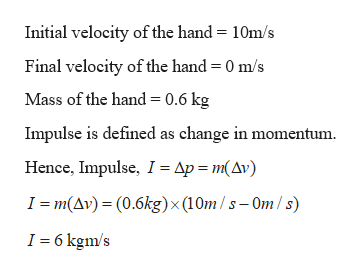# In tae-kwon-do, a hand is slammed down onto a target at a speed of 10 m/s and comes to a stop during the 3.0 ms collision. Assume that during the impact the hand is independent of the arm and has a mass of 0.60 kg. What are the magnitudes of the (a) impulse and (b) average force on the hand from the target?

Question

In tae-kwon-do, a hand is slammed down onto a target at a speed of 10 m/s and comes to a stop during the 3.0 ms collision. Assume that during the impact the hand is independent of the arm and has a mass of 0.60 kg. What are the magnitudes of the (a) impulse and (b) average force on the hand from the target?

check_circleExpert Solution
Step 1

Given:

Mass of the hand = 0.6 kg

Speed of the hand = 10 m/s

Time of impact = 3 ms

Step 2

Calculating the impulse:help_outlineImage TranscriptioncloseInitial velocity of the hand = 10m/s Final velocity of the hand = 0 m/s Mass of the hand = 0.6 kg Impulse is defined as change in momentum Hence, Impulse, I = Ap m(Av) I m(Av)(0.6kg)x (10m/s-Om/ s) I 6 kgm/s fullscreen
Step 3

Calculating the ave...

### Want to see the full answer?

See Solution

#### Want to see this answer and more?

Solutions are written by subject experts who are available 24/7. Questions are typically answered within 1 hour*

See Solution
*Response times may vary by subject and question
Tagged in

### Newtons Laws of Motion